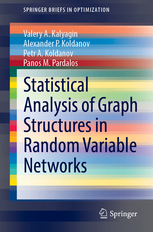• A
• A
• A
• ABC
• ABC
• ABC
• ąÉ
• ąÉ
• ąÉ
• ąÉ
• ąÉ
Regular version of the site

## Statistical Analysis of Graph Structures in Random Variable Networks

Springer, 2020.

This book studies complex systems with elements represented by random variables. Its main goal is to study and compare uncertainty of algorithms of network structure identification with applications to market network analysis. For this, a mathematical model of random variable network is introduced, uncertainty of identification procedure is defined through a risk function, random variables networks with different measures of similarity (dependence) are discussed, and general statistical properties of identification algorithms are studied. The volume also introduces a new class of identification algorithms based on a new measure of similarity and prove its robustness in a large class of distributions, and presents applications to social networks, power transmission grids, telecommunication networks, stock market networks, and brain networks through a theoretical analysis that identifies network structures. Both researchers and graduate students in computer science, mathematics, and optimization will find the applications and techniques presented useful.